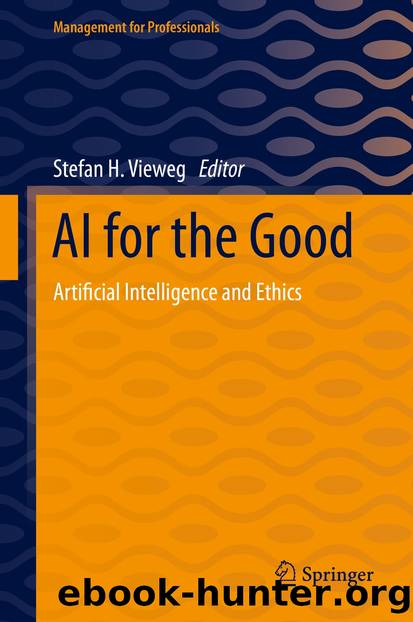# AI for the Good by UnknownAuthor:Unknown
Language: eng
Format: epub
ISBN: 9783030669133
Publisher: Springer International Publishing

To assess the accuracy of the different models, the median prediction has been taken as the naÃ¯ve baseline, which results in (due to the normalization from one hot encoding, results are directly in %):Median Baseline Mean Absolute Error

MAE = 3.7879%

Median Baseline Root Mean Square Error

RMSE = 4.8252%

The model initiation is configured as follows:model1 = LinearRegression() # no parametrization

model2 = ElasticNet(alpha=1.0, l1_ratio=0.5)

model3 = RandomForestRegressor(n_estimators=100)

model4 = ExtraTreesRegressor(n_estimators=100)

model5 = SVR(kernel='rbf', degree=3, C=1.0, gamma='auto')